# Colleges with the highest SAT scores in Florida

Top 10 colleges in Florida with the highest SAT scores
Looking for the colleges with the highest SAT scores in Florida? Well you're in luck! We've compiled a national college database and have created a list of the top 10 universities with the highest SAT scores in Florida. These are the schools whose applicants had the highest average SAT scores in Florida. And, since these tests are meant to determine academic prowess, they are arguably the schools with the most academically proficient students. You could even say these are the best colleges in Florida. We also include each college's ACT scores and acceptance rate so that you can see where you would have the easiest or hardest time getting in. Read on to find out more.

## University of Florida SAT scores

The average SAT score for University of Florida is 1360.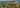The average SAT score of 1360 breaks down into:

• SAT math: 685

The average ACT score for University of Florida is 30 and their acceptance rate is 38.8%.

## University of Miami SAT scores

The average SAT score for University of Miami is 1355.The average SAT score of 1355 breaks down into:

• SAT math: 690

The average ACT score for University of Miami is 31 and their acceptance rate is 27.1%.

## New College of Florida SAT scores

The average SAT score for New College of Florida is 1324.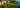The average SAT score of 1324 breaks down into:

• SAT math: 650

The average ACT score for New College of Florida is 29 and their acceptance rate is 76.8%.

## Florida State University SAT scores

The average SAT score for Florida State University is 1270.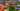The average SAT score of 1270 breaks down into:

• SAT math: 630

The average ACT score for Florida State University is 28 and their acceptance rate is 36%.

## University of Central Florida SAT scores

The average SAT score for University of Central Florida is 1259.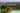The average SAT score of 1259 breaks down into:

• SAT math: 626

The average ACT score for University of Central Florida is 27 and their acceptance rate is 44.4%.

## Rollins College SAT scores

The average SAT score for Rollins College is 1252.The average SAT score of 1252 breaks down into:

• SAT math: 620

The average ACT score for Rollins College is 27 and their acceptance rate is 66.6%.

## University of South Florida SAT scores

The average SAT score for University of South Florida is 1250.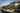The average SAT score of 1250 breaks down into:

• SAT math: 625

The average ACT score for University of South Florida is 27 and their acceptance rate is 42.7%.

## Florida Institute of Technology SAT scores

The average SAT score for Florida Institute of Technology is 1227.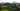The average SAT score of 1227 breaks down into:

• SAT math: 630

The average ACT score for Florida Institute of Technology is 27 and their acceptance rate is 63.5%.

## Stetson University SAT scores

The average SAT score for Stetson University is 1217.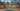The average SAT score of 1217 breaks down into:

• SAT math: 600

The average ACT score for Stetson University is 26 and their acceptance rate is 68.4%.

## Florida Southern College SAT scores

The average SAT score for Florida Southern College is 1214.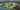The average SAT score of 1214 breaks down into:

• SAT math: 600